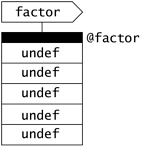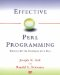# Item 31: Create lists of lists with references.

Before the introduction of references, Perl had no support for complex data types. Programmers had to resort to tricks in cases in which they needed to maintain structures like lists of lists. One common means was using strings together with split and join :

Factorize numbers , very inefficiently.

 ` @factor = ('') x 20; for (\$i = 2; \$i < 20; \$i++) { for (\$j = 2; \$j < \$i; \$j++) { if (\$i % \$j == 0) { \$factor[\$i] .= "\$j "; } } } ` Build up strings like "2 3 4 6" . ` for (\$i = 2; \$i < 20; \$i++) { print("\$i: \$factor[\$i]\n"); } ` 12: 2 3 4 6 13: etc.

References give you a way to create lists of lists and other nested structureone that is much more efficient and elegant than repeatedly disassembling and reassembling strings.

Here, for example, are some lists of lists:

 ` @a = ( [1, 2], [3, 4] ); print \$a; ` An array of refs to arrays. Prints 3 . ` \$a = [ [1, 2], [3, 4] ]; ` A ref to an array of refs to arrays. Also prints 3 . ` print \$a->; `

Note that the type of parentheses/brackets you use must match the type of structure you are creating (see Item 32).

You can also create these structures dynamically. Here is the factorization example rewritten to use references:

Still algorithmically awful , but at least it uses references.

 ` for (\$i = 2; \$i < 20; \$i++) { for (\$j = 2; \$j < \$i; \$j++) { if (\$i % \$j == 0) { push @{\$factor[\$i]}, \$j; } } } ` Build up arrays instead of strings. ` for (\$i = 2; \$i < 20; \$i++) { print("\$i: @{\$factor[\$i] []}\n"); } ` Writing [] inside the array reference avoids warnings under -w .

In this example, @factor is an array of references to arrays. We do not have to initialize it at all, because it is created automatically by auto-vivification (see Item 30) as we try to add elements to it.

Let's look at this more closely. Assume that @factor is undefined to start with. The very first time we encounter the statement containing the push operator will be when \$i is 4 and \$j is 2 . Perl first tries to access \$factor . It's not there, so as usual, Perl creates an array of the requisite size and fills it with undef values. Now, @factor is defined:Perl now tries to push the value 2 onto the list @{\$factor} . The syntax suggests that \$factor should be a reference to an array, and so Perl auto-vivifies an empty anonymous array and puts a reference to it in \$factor :Finally, Perl pushes the value 2 onto the newly created list.

The principles demonstrated in this example can be used to create other structures, like multidimensional arrays and hashes of hashes:

 ` \$d3 = 5; \$d3->-> = 5; \${\${\$d3}} = 5; ` 3-d array on the fly. Same, explicit arrows. Ugly canonical syntax. ` \$d3-> = 5; \${\${\$d3->}} = 5; \${\${\${\$d3}}} = 5; ` Similar, but starting with a ref. Pretty ugly without the arrows, eh? ` \$course{'CS'}{'101'} = 'Intro to Programming'; ` A hash of hashes starting with %course .

For more ways to create and manipulate nested structures, see Item 35.Effective Perl Programming: Writing Better Programs with Perl
ISBN: 0201419750
EAN: 2147483647
Year: 1996
Pages: 116

Similar book on Amazon